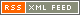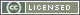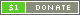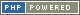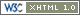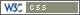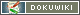# Arithmetic Operators

Arithmetic Operators perform basic arithmetic operations on the terms specified. There are different arithmetic operations available for each type of value.

Symbol Type Name Description Example
Number
+ Infix Addition Adds the right term to the left term to give the sum ` a + b `
- Infix Subtraction Takes the right term away from the left term to give the difference ` a - b `
* Infix Multiplication Multiplies the left term by the right term to give the product ` a * b `
/ Infix Division Divides the left term by the right term to give the quotient ` a / b `
% Infix Modulus Divides the left term by the right term and gives the remainder ` a % b `
** Infix Exponentiation Raises the left term to the power specified by the right term ` a ** b `
String
~ Infix Concatenation Joins the right term to the end of the left term ` a ~ b `
~* Infix Repetition Repeats the left term by the number specified by the right term ` a ~* b `
Array
@ Infix Merge Joins the right term to the end of the left term ` a @ b `
@* Infix Repetition Repeats the left term by the number specified by the right term ` a @* b `

Each Operator will evaluate the terms provided as appropriate to the Operator value type - for instance, performing a numerical addition on two Strings will convert the Strings to Numbers first.